## Ti NSpire CX: Implicit Differentiation – Step by Step

Using Calculus Made Easy on the TiNSpire CX at  https://www.tinspireapps.com/?a=CME

choose IMPLICIT DIFFERENTIATION in the menu , then enter  the equation of a

given curve as shown below:The Implicit Differentiation process continues until step 5)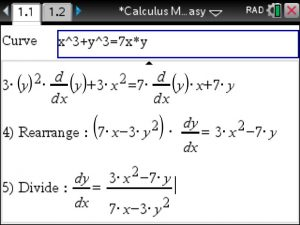VOILA ! easy as pie! The handy TiNspire CX can not only Implicit Differentiation Step by Step but can do ALL

of Calculus Step by Step!

Posted on Categories calculus, tinspirecx

## ▷[Solved] Compute Limits in Calculus – Step by Step – using the TiNspire CX CAS

In your Calculus class you will be asked to compute limits. You should know that there is a variety of techniques to figure out limits such as via Factoring, via Common Denominator and of course via L’Hopital rule (which we covered in earlier posts already) . All these techniques can be applied using the Calculus Made Easy app , just navigate to Limits in the Menu as seen below:When selecting the menu option 4: Limit via Factoring you enter your function and the x value that you are approaching. Now lean back and observe the steps to arrive at the limit. Calculus all of a sudden got easy! See below.Another common method to compute Limits is via Common Denominator of the numerator. Read the sentence again to digest it better 😉

The tricky part is to enter the two fractions in the numerator properly, extra set of parentheses are needed here. See belowThe steps to compute the limits are displayed below: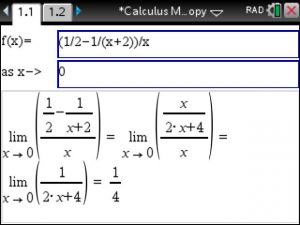These Step by Step Limit computation methods using your TiNspire CX CAS will allow you to master your Calculus class.

Posted on Categories calculus, Limits, solver, tinspirecx

## ▷Solved: Volume of Solid of Rotation by Washer Method using the TiNspire CX

Computing the Volume of a solid of rotation using the Washer or Shell Method

can easily be performed using TiNSpire’s Calculus Made Easy app as follows.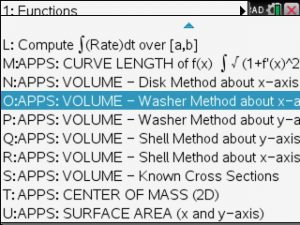After selecting the Washer Method about x-axis just enter the upper U and lower function L

(dont worry in case you swap them : the answer will be correct except it will have a minus sign with it. )

and the interval bounds a and b as shown in belows image: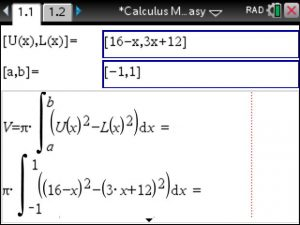You can see the general washer method formula and how it is applied to you specific problem.

Additionally, you will see the antiderivative (if it is exists), the exact and finally the correct decimal answer.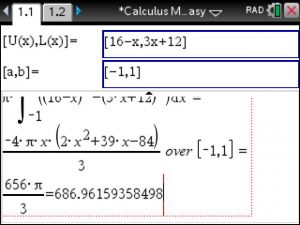Finding the volume of solids of rotation and with known cross sections are just a few integral applications included in the Calculus Made Easy app of your TiNspire. Other included applications are surface area of solids, curve lengths, center of math, improper integrals, etc

Best of all Calculus Made Easy can be used for 3 courses of Calculus: Calculus I, II and III, the latter being a typical Multivariable Calculus course which involves partial derivatives and multiple integrals.

Posted on Categories calculus, tinspirecx

## ▷Riemann Sums – Step by Step – with the TiNSpire CX CAS: Calculus Made Easy

Say you need to compute Riemann Sums at selected points over a given interval. And you do have your trusty TiNspire CX on you. Then start Calculus Made Easy , go to option 1 J in the menu and enter

1. the given function
2. the given interval
3. the selected x-values c1, c2, ..

as shown in image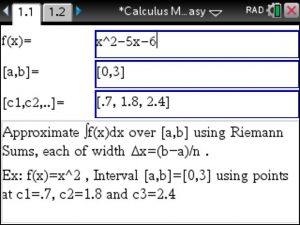Then each Riemann rectangle is computed step by step as shown below :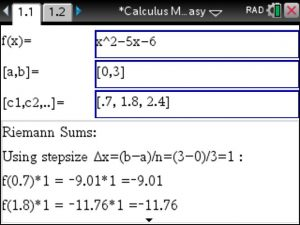Lastly, view the final Riemann Sum and compare it to the exact definite integral. Pretty close 😉Posted on Categories calculus, Integral

## ▷Integration by Parts – Step by Step – using the Tinspire CX : Calculus Made Easy

Integration by Parts with steps is easily done using Calculus Made Easy (download : www.tinspireapps.com ) under the Integration tab.

Enter your function as f(x) and your choices of u and v’ below.

See example in image:Answer appears automatically with steps involved.Steps for Integration and Differentiation and all other Calculus topics are included in the Calculus Made Easy app.

Posted on Categories calculus, Integral

## ▷Relative Rate of Change using the TiNspire CX CAS

To find the Relative Rate of Change using the TiNSpire CX CAS open up Calculus Made Easy from www.TiNspireCX.com , then go menu item “Derivatives” and select “Relative Rate of Change”. Enter the given Function and Voila, the Relative Rate of Change shows immediately, Very simple to use !!!

Remember that Relative Rate of Change in Calculus is the Derivative of a Function divided by the Function itself. Here is an example:

f(x) = 4x + exp(7x) , then f'(x) = 4 + 7*exp(7x) ,

Thus , Relative Rate of Change of f =

(4 + 7*exp(7x) ) / (4x + exp(7x) )

Posted on Categories calculus

## Taylor and McLaurin Series Step by Step on the TiNspire CX – Calculus Made Easy

Finding Taylor Series and MacLaurin Series Step by Step using the TiNspire is as easy as pie:

Say you are asked to find the Taylor Series centered at a=0 up to degree n=3 (really a MacLaurin series as the center is at 0 )

So plug into Calculus Made Easy option 7 D as follows :The derivatives are taken in order to compute the coefficients for each term up to degree 3.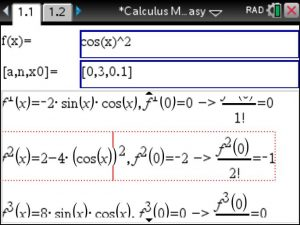The final answer is of degree 2 as the coefficient for the 3rd degree term is 0.Additionally, other series topics such as interval of convergence and alternating series and Lagrange errors can be found as well using Calculus Made Easy at https://tinspireapps.com/?a=cme

Posted on Categories calculus, Taylor Series

## Finding Critical Points on the TiNspire CX CAS – using Calculus Made Easy

Finding Critical Points and also a relative & absolute Minimum or Maximum is easily done using the

Calculus Made Easy APP at www.TiNspireApps.com  . Simply select “Critical Points” in the Menu.Here, you can either enter the given function f(x) in the top box or
enter its given derivative in the bottom box.  We enter f(x) in below’s example.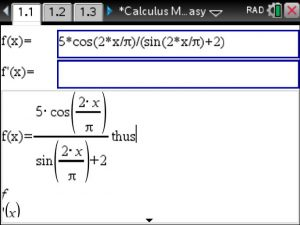Then, the derivative f'(x) is computedand simplified to a more compact expression: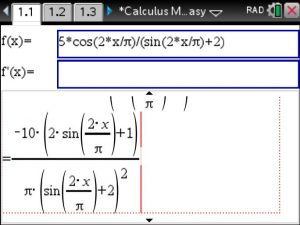finding its zeros yields the following x values of the critical points.

Since we entered a periodic trigonometric function f(x)
we obtain infinitely many critical points of the format
shown below. Note that n87 represents a constant which
is usually denoted as K in textbooks.Besides finding critical points, Maximum and Minimum values
you will also be able to find saddle points and inflection points under this menu option.

## TiNspire Integration via U-Substitution Solver – Step by Step – using Calculus Made Easy

Here are great news: Now Calculus Made Easy for the TiNspire CX CAS  offers you two different ways to

perform Integration using U-Substitution.

The first way is the fully automated: Just plug in your given function as seen below and steps and answer are displayed.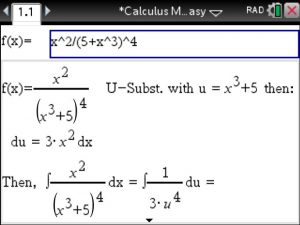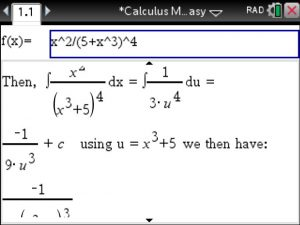The second way requires your input on the choice of u. You may wonder what is

point if the fully automated version can do this already?  It is called LEARNING:

U Substitutions are not unique: Different choices of U may lead to the same solution.

Check out below’s example: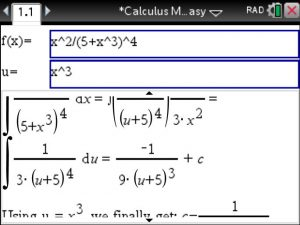Check out another choice of U, it leads to the same solution.

Our Made Easy APPS aim at empowering students and this feature allows you guys to
learn about U-Substitution in a way you likely will not in your classroom. So enjoy this feature.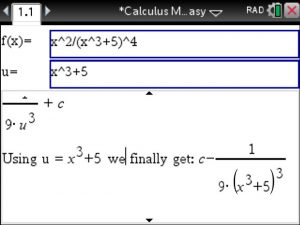As usual, updates are free forever.

## Shell Method to find Volume of Solid when rotating about x-axis – TiNspire CAS CX in Calculus Made Easy

your teacher wants you to find Volume of Solid when rotating an enclosed area about x-axis using the cylindrical shell method
You have a TiNspire CAS CX and Calculus Made Easy from www.TinspireApps.com ? You are all set, just check the images!